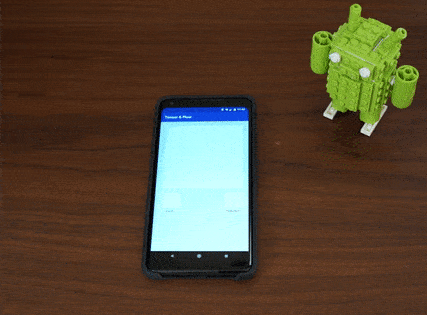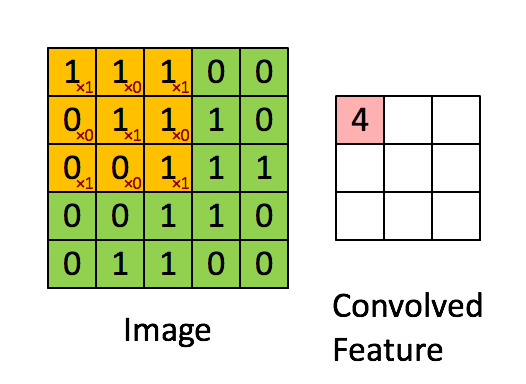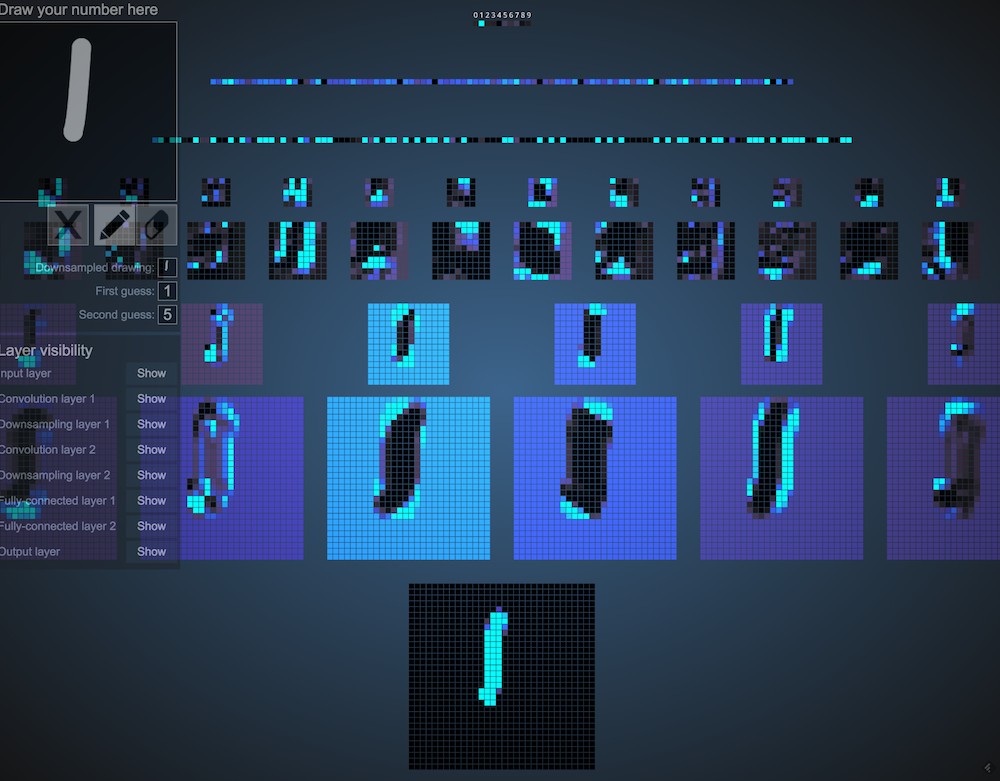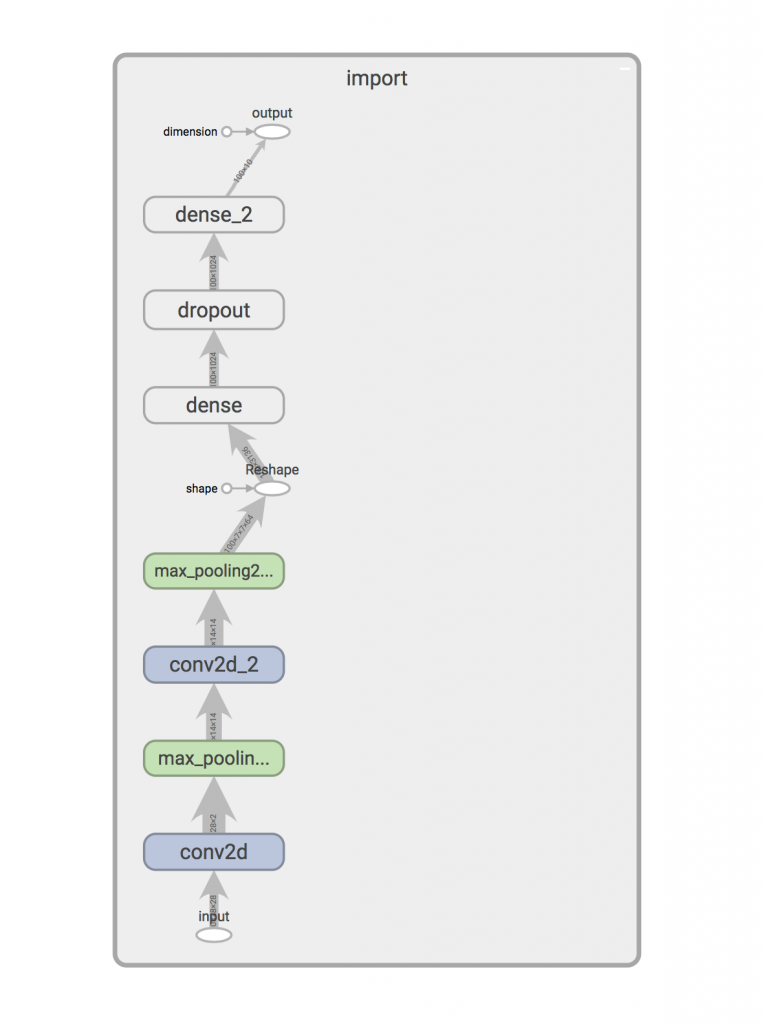Many popular apps on both Android and iOS make extensive use of on-device Machine Learning. Apps like Inbox by Gmail or Siri make heavy use of on device machine learning because it’s faster and does a better job of protecting a user’s privacy. iOS and Android both have proper API support for using on device, neural networks for prediction purposes. On both platforms you can either wire up your own neural network or use a higher level framework like TensorFlow to do the heavy lifting for you.

In Tensor & Flow we will explore the specifics of what is needed to do to deploy a machine learning model to an Android app. I will be using TensorFlow Mobile in Part 1.Tensor & Flow demo app on Android

## Training a Neural Network

The very first step on this journey is training a neural network that I can deploy. There are plenty of tutorials that walk aspiring machine learning engineers through building models that can classify flowers, identify objects in pictures, detect spam, and even apply filters to pictures. I chose a rather accessible tutorial, building a model to recognize handwritten numbers.

A Guide to TF Layers: Building a Convolutional Neural Network walks us through the entire process configuring and training a neural network to recognize handwritten characters. This guide walks us neural network configuration, downloading the dataset used for training, and the training process.

The first step is configuring our neural network.

### The Neural Network

The MNIST tutorial trains a Convolutional Neural Network (CNN) to recognize handwritten numbers.Feature extraction using convolution Source

A CNN is comprised of several different layers:

• Convolutional layers, use convolution operations to extract features from images.
• Pooling layers, down sample the images, which reduces processing time and increases training and inference performance.
• Dense layers, predict a class using the features extracted in the convolutional layers.

Before continuing, I encourage you to make your way over to the Data Science Blog where Ujjwal Karn has written up a very intuitive blog post aptly named “An Intuitive Explanation of Convolutional Neural Networks”. Once you have finished the blog post, visit 2D Visualization of a Convolutional Neural Network for a cool demonstration of a CNN in action.2D Visualization of a CNN

Some more specifics about the neural network in this example, the input layer is a one to one mapping of the size of the input data. The MNIST dataset contains tens of thousands of handwritten number samples and labels. Each sample is a monochrome image of a handwritten digit, 28 pixels x 28 pixels. An image is a 2-dimension array, containing of pixel data, meaning our input layer has 784 input nodes (28 x 28 = 784).

The output layer, a Logits layer, that emits our predictions as raw values. The network uses several additional functions to convert this raw data into a prediction and probability (for training).

### Training & Prepping for Integration

The process for training and integrating a neural network model into an Android app resembles:

1. Train the neural network.
2. Freeze & optimize the TensorFlow graph for inference.
3. View the neural network model in TensorBoard. (optional)
4. Import the optimized graph into our Android project.

Getting everything setup to do the training can be more difficult than the actual training depending on your computing platform. My setup:

• MacBook Pro (2015) running MacOS 10.13
• IDE: PyCharm, made this easier by auto-importing Python dependencies and providing code debugging capabilities
• Python 2.7.13
• TensorFlow 1.5
• Android Studio 3.0.1

Our guide “A Guide to TF Layers” walks us through setting up our neural network and training. After a few passes through the guide, I made one tweak that made integration into an Android app a bit easier, I gave explicit names to my input and output layers, “input” & “output”, respectively. I did this after spending a few hours attempting to figure out on my own. If you do not name the layers in your neural network, they are given default names. You’ll need to open your trained graph in TensorBoard to determine the names of your layers.

We will end up with this Python script:

``````from __future__ import absolute_import
from __future__ import division
from __future__ import print_function

# Imports
import numpy as np
import tensorflow as tf

tf.logging.set_verbosity(tf.logging.INFO)

def cnn_model_fn(features, labels, mode):
"""Model function for CNN."""
# Input Layer
input_layer = tf.reshape(features["x"], [-1, 28, 28, 1], name="input")

# Convolutional Layer #1
conv1 = tf.layers.conv2d(
inputs=input_layer,
filters=32,
kernel_size=[5, 5],
activation=tf.nn.relu)

# Pooling Layer #1
pool1 = tf.layers.max_pooling2d(inputs=conv1, pool_size=[2, 2], strides=2)

# Convolutional Layer #2 and Pooling Layer #2
conv2 = tf.layers.conv2d(
inputs=pool1,
filters=64,
kernel_size=[5, 5],
activation=tf.nn.relu)
pool2 = tf.layers.max_pooling2d(inputs=conv2, pool_size=[2, 2], strides=2)

# Dense Layer
pool2_flat = tf.reshape(pool2, [-1, 7 * 7 * 64])
dense = tf.layers.dense(inputs=pool2_flat, units=1024, activation=tf.nn.relu)
dropout = tf.layers.dropout(
inputs=dense, rate=0.4, training=mode == tf.estimator.ModeKeys.TRAIN)

# Logits Layer
logits = tf.layers.dense(inputs=dropout, units=10)

predictions = {
# Generate predictions (for PREDICT and EVAL mode)
"classes": tf.argmax(input=logits, axis=1, name="output"),
# Add `softmax_tensor` to the graph. It is used for PREDICT and by the
# `logging_hook`.
"probabilities": tf.nn.softmax(logits, name="softmax_tensor")
}

if mode == tf.estimator.ModeKeys.PREDICT:
return tf.estimator.EstimatorSpec(mode=mode, predictions=predictions)

# Calculate Loss (for both TRAIN and EVAL modes)
loss = tf.losses.sparse_softmax_cross_entropy(labels=labels, logits=logits)

# Configure the Training Op (for TRAIN mode)
if mode == tf.estimator.ModeKeys.TRAIN:
train_op = optimizer.minimize(
loss=loss,
global_step=tf.train.get_global_step())
return tf.estimator.EstimatorSpec(mode=mode, loss=loss, train_op=train_op)

# Add evaluation metrics (for EVAL mode)
eval_metric_ops = {
"accuracy": tf.metrics.accuracy(
labels=labels, predictions=predictions["classes"])}
return tf.estimator.EstimatorSpec(mode=mode, loss=loss, eval_metric_ops=eval_metric_ops)

def main(unused_argv):
# Load training and eval data
train_data = mnist.train.images  # Returns np.array
train_labels = np.asarray(mnist.train.labels, dtype=np.int32)
eval_data = mnist.test.images  # Returns np.array
eval_labels = np.asarray(mnist.test.labels, dtype=np.int32)

# Create the Estimator
mnist_classifier = tf.estimator.Estimator(model_fn=cnn_model_fn, model_dir="/tmp/mnist_convnet_model")

# Set up logging for predictions
tensors_to_log = {"probabilities": "softmax_tensor"}
logging_hook = tf.train.LoggingTensorHook(tensors=tensors_to_log, every_n_iter=50)

# Train the model
train_input_fn = tf.estimator.inputs.numpy_input_fn(
x={"x": train_data},
y=train_labels,
batch_size=100,
num_epochs=None,
shuffle=True)
mnist_classifier.train(
input_fn=train_input_fn,
steps=20000,
hooks=[logging_hook])

# Evaluate the model and print results
eval_input_fn = tf.estimator.inputs.numpy_input_fn(
x={"x": eval_data},
y=eval_labels,
num_epochs=1,
shuffle=False)
eval_results = mnist_classifier.evaluate(input_fn=eval_input_fn)
print(eval_results)

if __name__ == "__main__":
tf.app.run()
``````

It configures our neural network in `cnn_model_fn`. Training happens in `main`. During our training step, we download the MNIST dataset, which is already broken up into a training and evaluation chunks. When training a neural network, you want to be sure you make a subset of your training data available for evaluation purposes. This allows you to test the accuracy of your neural network as training progresses. This can also prevent you from overfitting your neural network to the training data.

Starting training is as easy using the command `python train_cnn.py`. Depending on the hardware configuration of your computer, training will take anywhere from minutes to hours. This script is configured to train the network for 20,000 iterations. While your training script is running, you’ll periodically see output that shows the progress of the training process.

``````INFO:tensorflow:global_step/sec: 2.75874
INFO:tensorflow:probabilities = [[ 0.10167542  0.10189584  0.10309957  0.11525927  0.09659223  0.08847987
0.09406721  0.10499229  0.093654    0.10028425]
[ 0.10425898  0.11098097  0.10286383  0.09657481  0.10871311  0.08486023
0.09235432  0.09499202  0.10640075  0.09800103]
[ 0.1033088   0.11629853  0.11034065  0.0981971   0.08924178  0.09668511
0.10001212  0.09568888  0.08589367  0.10433336]
[ 0.10667751  0.10386481  0.09242702  0.11075728  0.08897669  0.09205832
0.10070907  0.10779921  0.08927511  0.10745502]
...
``````

It shows the rate of training and an array of probabilities of that sample image being a number. For example:

``````[ 0.00001972  0.00000233  0.00022174  0.00427989  0.00001842  0.97293282
0.00000114  0.00013626  0.00584014  0.01654756]
``````

There looks to be a 97.3% probability that this sample image is the number represented by this index (5 or 6 depending on the starting index). These values become more certain as training continues. The neural network is improving its ability to identify the handwritten digits.

Compare these probabilities at the beginning of training:

``````[ 0.1033088   0.11629853  0.11034065  0.0981971   0.08924178  0.09668511
0.10001212  0.09568888  0.08589367  0.10433336]
``````

With these, near the end:

``````[ 0.00000006  0.0000001   0.00000017  0.00000019  0.99616736  0.00000038, 0.00000154  0.00000558  0.00001187  0.00381267]
``````

You’ll notice that the network is becoming more accurate with it’s predictions.

Once training has finished, it will test the neural network against a second dataset of labeled images. This is used to calculated an accuracy of the trained network.

``````INFO:tensorflow:Saving dict for global step 20000: accuracy = 0.9708, global_step = 20000, loss = 0.0991706
``````

As of step 20000, our neural network has a prediction accuracy of 97.8%! A bit about the `loss` value. The goal when training a neural network is to minimize the loss, or the difference between the predicted value and the actual value. Generally, a neural network with a lower amount of `loss` will give more accurate predictions.

Now that training is complete, we end up with a bunch of files in `/tmp/mnist_convnet_model`:

``````checkpoint
eval
events.out
graph.pbtxt
model.ckpt-15199.data-00000-of-00001
model.ckpt-15199.index
model.ckpt-15199.meta
model.ckpt-20000.data-00000-of-00001
model.ckpt-20000.index
model.ckpt-20000.meta
``````

The files we are concerned with for the next steps are:

``````graph.pbtxt                           // graph definition file, human readable protobuf format
model.ckpt-20000.data-00000-of-00001  // stores the values of variables from our graph
model.ckpt-20000.index                // identifies the checkpoint / training step
model.ckpt-20000.meta                 // stores the structure of the graph
``````

We need to do a bit of massaging before integrating them into an Android app. We will:

1. Freeze our graph and values. This stores the neural network and tensor values in one binary graph definition file (.pb), instead of separate files.
2. Optimize our graph for inference. After the training step finishes, our graph contains nodes that were used during training. These nodes are not needed when doing inferencing. This optimization will remove these extra nodes and tensors. This reduces the size of our graph and also reduces the chance that we’ll encounter an operation in our graph that isn’t supported by TensorFlow Mobile.

#### Freezing

Freezing our graph is as easy as executing a Python script thats available in the TensorFlow repository.

Execution looks like:

``````python freeze_graph.py \
--input_graph=/tmp/mnist_convnet_model/graph.pbtxt \
--input_binary=false \
--input_checkpoint=/tmp/mnist_convnet_model/model.ckpt-20000 \
--output_graph=/tmp/mnist_convnet_model/frozen_graph.pb \
--output_node_names=output \
``````

When this script has completed, you’ll see a new file `frozen_graph.db` in `/tmp/mnist_convnet_model`. It contains our graph definition and network values.

#### Optimizing for Inference

Now, we optimize our neural network for inferencing. There’s also a script that is available in the TensorFlow repository. Optimizing for inference removes parts of the graph that are only needed for training.

``````python optimize_for_inference.py \
--input=/tmp/mnist_convnet_model/frozen_graph.pb \
--output=/tmp/mnist_convnet_model/optimized_graph.pb \
--input_names=input \
--output_names=output
``````

When this script is finished, you’ll see a new file `optimized_graph.db` in `/tmp/mnist_convnet_model`. This is the final state of our neural network model.

This is a TensorBoard view our frozen and optimized graph.If you want to visualize your optimized graph with TensorBoard, check out How to inspect pretrained TF model.

We are now ready to begin using this in an Android project.

### Integrating into an Android App

Deploying a trained TensorFlow neural network model is a relatively task.

#### Adding the TensorFlow Mobile dependency

Add the TensorFlow Mobile dependency to the `build.gradle` in the `app/` folder, then sync the project’s Gradle dependencies.

``````implementation "org.tensorflow:tensorflow-android:1.5.0"
``````

The class we are going to use to interact with our model, provided by TensorFlow Mobile, is `TensorFlowInferenceInterface`. It provides several methods for loading our model, feeding new data to the network, running inference, and extracting a prediction.

Copy your optimized graph to your Android project. It should be copied to `src/main/assets`. `TensorFlowInferenceInterface` will load the model from this folder in it’s constructor.

#### Some architecture

Our app will allow the user to draw a number with their finger. We will convert their drawing into a bitmap and pass that to our neural network for prediction. Recognizing this, the very first thing I will do is create a `Classifier` interface.

``````interface Classifier {
fun predict(input: IntArray): Int
fun close()
}
``````

I am choosing to create an interface so that I can easily create more than one implementation of a Classifier. One using TensorFlow Mobile and one using TensorFlow Lite (in Part 2).

#### Using TensorFlowInferenceInterface

As stated before `TensorFlowInferenceInterface` is how we will be interacting with our trained network.

Let’s implement our `Classifier` interface by writing `TFMobileClassifier`.

``````class TFMobileClassifier(context: Context,
modelFilename: String,
private val inputName: String,
private val inputDimensions: Pair<long , Long>,
private val outputName: String,
private val outputSize: Int) : Classifier {

override predict(input: IntArray): Int {
TODO()
}

override close() {
TODO()
}
}
``````

Our `TFMobileClassifier` has a constructor with 6 arguments. The `Context` is used to access files via `AssetManager`. The remaining arguments specify our model file and input and output node specifications.

Instantiating a `TFMobileClassifier`.

``````val classifier: Classifier = TFMobileClassifier(this,
modelFilename = "file:///android_asset/optimized_graph.pb",
inputName = "input",
inputDimensions = Pair(28, 28),
outputName = "output",
outputSize = 100)
``````

Let’s create our `TensorFlowInferenceInterface`.

private val assetManager = context.assetManager private val inferenceInterface = TensorFlowInferenceInterface(assetManager, modelFilename)

Now that we have have a `TensorFlowInferenceInterface`, let’s start using it by implementing `predict()`.

``````override fun predict(input: FloatArray) {
// 1) create an array to store our predictions
val predictions = LongArray(100)

// 2) feed our data into input layer of our neural network
inferenceInterface.feed(inputName, floatInput, 1, inputDimensions.first, inputDimensions.second, 1)

// 3) run inference between the input and specified output nodes
inferenceInterface.run(arrayOf(outputName))

// 4) fetch the predictions from the specified output node
inferenceInterface.fetch(outputName, predictions)

// 5) tabulate our predictions and return the most probable
return processPredictions(predictions)
}
``````

A few things to talk about here:

1. Our output node emits 100 values, so we need to store them in an array that contains at least 100 elements
2. Our input data array size must equal the value when you compute the total elements in a X * Y * Z array. For example, our neural network uses 28 x 28 monochrome images. Our dimensions are going to be: 28 x 28 x 1. This means our input data array should contain 784 values.
3. When running inference, we need to specify the name of the output node where inference will end.
4. After inference has completed, we will store our results in the 100 element predictions array. This particular neural network returns an array containing 100 predictions given the input data. Going back to our Python training script, we trained our network in with batch_size = 100. This means, even though we feed the neural network a single image, it will give us 100 predictions on what it thinks the user has drawn.
5. Because we have 100 predictions, we need to count the occurrence of each prediction, then return the digit that was the predicted the most. We will use this value as our prediction.

Our implemented `TFMobileClassifier`.

``````package com.emuneee.tensorandflow.classifier

import android.content.Context
import android.content.res.AssetManager
import org.tensorflow.contrib.android.TensorFlowInferenceInterface
import timber.log.Timber
import java.util.*
import kotlin.Comparator

/**
* Created by evan on 2/28/18.
*/
class TFMobileClassifier(context: Context,
modelFilename: String,
private val inputName: String,
private val inputDimensions: Pair<long , Long>,
private val outputName: String,
private val outputSize: Int) : Classifier {

private val assetManager: AssetManager = context.assets
private val inferenceInterface = TensorFlowInferenceInterface(assetManager, modelFilename)

override fun predict(input: IntArray): Int {
val floatInput = input.map { it.toFloat() }
.toFloatArray()
// 1) create an array to store our predictions
val predictions = LongArray(outputSize)

// 2) feed our data into input layer of our neural network
inferenceInterface.feed(inputName, floatInput, 1, inputDimensions.first, inputDimensions.second, 1)

// 3) run inference between the input and specified output nodes
inferenceInterface.run(arrayOf(outputName))

// 4) fetch the predictions from the specified output node
inferenceInterface.fetch(outputName, predictions)

// 5) tabulate our predictions and return the most probable
return processPredictions(predictions)
}

private fun processPredictions(predictions: LongArray): Int {
val counts = predictions.toTypedArray()
.groupingBy { it }
.eachCount()
val predictionSet = TreeSet<pair <Long, Int>>(Comparator</pair><pair <Long, Int>> { o1, o2 -> o2!!.second.compareTo(o1!!.second) })
counts.toList()
.forEach { pair -> predictionSet.add(pair) }
val pair = predictionSet.first()
Timber.d("Selecting \${pair.first} @ \${(pair.second / 100.0) * 100}% confidence")
return pair.first.toInt()
}

override fun close() {
inferenceInterface.close()
}
}
``````

#### Using the Classifier

Now that we have implemented a `Classifier`, it’s time to build some UI that allows the user to submit data with their fingertips. For brevity’s sake, I’m going to pass over a lot of the pure Android concepts, like layouts, and click listeners, etc. Our user interface has 3 components:

1. We have a custom `CanvasView` that allows the user to user their fingertips to draw on a `Canvas`. When the user has finished drawing on the `CanvasView` it will emit a bitmap that represents the user’s drawing via a `CanvasView.DrawListener`
2. We’ll have an `ImageView` that resembles actual data submitted to the neural network.
3. Finally, we’ll have a `TextView` that displays the prediction.

Before we continue, we will need to address an issue. We’ll need to convert the user input to data format that resembles an image from the MNIST dataset. This is critical because the closer the data resembles the original training data, the more accurate our predictions. The MNIST training data set is filled with 28x28 monochrome images where for a given pixel, the values range from 0 (white) to 255 (black).

Here is how we convert the bitmap from our `CanvasView` to a monochrome, 28x28 bitmap:

``````private fun toMonochrome(bitmap: Bitmap): Bitmap {
// scale bitmap to 28 by 28
val scaled = Bitmap.createScaledBitmap(bitmap, 28, 28, false)

// convert bitmap to monochrome
val monochrome = Bitmap.createBitmap(28, 28, Bitmap.Config.ARGB_8888)
val canvas = Canvas(monochrome)
val ma = ColorMatrix()
ma.setSaturation(0f)
val paint = Paint()
paint.colorFilter = ColorMatrixColorFilter(ma)
canvas.drawBitmap(scaled, 0f, 0f, paint)

val width = monochrome.width
val height = monochrome.height

val pixels = IntArray(width * height)
monochrome.getPixels(pixels, 0, width, 0, 0, width, height)

// Iterate over height
for (y in 0 until height) {

for (x in 0 until width) {
val pixel = monochrome.getPixel(x, y)
val lowestBit = pixel and 0xff

if (lowestBit < 128) {
monochrome.setPixel(x, y, Color.BLACK)
}
else {
monochrome.setPixel(x, y, Color.WHITE)
}
}
}
return monochrome
}
``````

The output from `toMonochrome()` is used to give the user an idea of what the input to the neural network looks like. It’s also converted to a format suitable for inference:

``````private fun formatInput(bitmap: Bitmap): IntArray {
val pixels = IntArray(bitmap.width * bitmap.height)
var i = 0

for (y in 0 until bitmap.height) {

for (x in 0 until bitmap.width) {
pixels[i++] = if (bitmap.getPixel(x, y) == Color.BLACK) 255 else 0
}
}
return pixels
}
``````

We do two things here. First we flatten our 28x28 bitmap into a 784 element integer array. Finally, we convert each pixel value to either `0` or `255` if the pixel value is white or black, respectively.

Our `MainActivity.kt` looks like:

``````package com.emuneee.tensorandflow

import android.graphics.*
import android.support.v7.app.AppCompatActivity
import android.os.Bundle
import kotlinx.android.synthetic.main.activity_main.*
import android.graphics.Bitmap
import com.emuneee.tensorandflow.classifier.Classifier
import com.emuneee.tensorandflow.classifier.TFMobileClassifier
import com.emuneee.tensorandflow.view.CanvasView
import timber.log.Timber

class MainActivity : AppCompatActivity() {

private val classifier: Classifier by lazy {
TFMobileClassifier(this,
modelFilename = "file:///android_asset/optimized_graph.pb",
inputName = "input",
inputDimensions = Pair(28, 28),
outputName = "output",
outputSize = 100)
}

override fun onCreate(savedInstanceState: Bundle?) {
super.onCreate(savedInstanceState)
setContentView(R.layout.activity_main)
Timber.plant(Timber.DebugTree())

canvas.drawListener = object: CanvasView.DrawListener {
override fun onNewBitmap(bitmap: Bitmap) {

// convert the drawing to a 28x28 monochrome image
val monochrome = toMonochrome(bitmap)

// set the nn input image

// convert the data to something that resembles the MNIST training data set
val inputData = toIntArray(monochrome)

// predict
val pred = classifier.predict(inputData)
runOnUiThread { prediction.text = pred.toString() }

}).start()
}
}
}

override fun onDestroy() {
super.onDestroy()
classifier.close()
}

/**
* Converts a Bitmap to a 28 x 28 monochrome bitmap
*/
private fun toMonochrome(bitmap: Bitmap): Bitmap {
// scale bitmap to 28 by 28
val scaled = Bitmap.createScaledBitmap(bitmap, 28, 28, false)

// convert bitmap to monochrome
val monochrome = Bitmap.createBitmap(28, 28, Bitmap.Config.ARGB_8888)
val canvas = Canvas(monochrome)
val ma = ColorMatrix()
ma.setSaturation(0f)
val paint = Paint()
paint.colorFilter = ColorMatrixColorFilter(ma)
canvas.drawBitmap(scaled, 0f, 0f, paint)

val width = monochrome.width
val height = monochrome.height

val pixels = IntArray(width * height)
monochrome.getPixels(pixels, 0, width, 0, 0, width, height)

for (y in 0 until height) {

for (x in 0 until width) {
val pixel = monochrome.getPixel(x, y)
val lowestBit = pixel and 0xff

if (lowestBit < 128) {
monochrome.setPixel(x, y, Color.BLACK)
}
else {
monochrome.setPixel(x, y, Color.WHITE)
}
}
}
return monochrome
}

/**
* Converts a bitmap to a flattened integer array
*/
private fun toIntArray(bitmap: Bitmap): IntArray {
val pixels = IntArray(bitmap.width * bitmap.height)
var i = 0

for (y in 0 until bitmap.height) {

for (x in 0 until bitmap.width) {
pixels[i++] = if (bitmap.getPixel(x, y) == Color.BLACK) 255 else 0
}
}
return pixels
}
}
``````

That’s it! We have trained a neural network to recognize handwritten digits using TensorFlow, then successfully deployed it via an Android app.Checkout all the code, scripts, and model on GitHub.

🧇